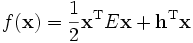# Numb3rs 323: Money for Nothing

In this episode, Charlie uses two algorithms to help him solve problems.

## Dijkstra's Algorithm

Edsger Dijkstra (1930-2002) was a Dutch computer scientist. He was instrumental in developing many of the ideas behind modern programming languages. Despite this, he is most famous for a relatively simple algorithm. Suppose we are given a graph G which is comprised of vertices V, edges E, and a weight function W. W takes edges as inputs and outputs a non-negative number. The usual interpretation of this scenario is that G is a map of highways: each vertex is a major city, each edge is a highway, and the weight of each edge is how long it takes to travel from one vertex to the other. Starting with a vertex s, Dijkstra's algorithm determines the weight of "lightest" path from s to every vertex in the graph.

The algorithm works as follows:

1. Fix a starting vertex s.
2. For each vertex x, create a function h(x) by defining h(x) to be infinity if x is not s and to be zero if x is s. In reality infinity could be picked as a very large number - say larger than the weight of heaviest edge times the number of vertices.
3. Let s=s0. Set S0={s0}
4. Find the vertex adjacent to s so that the edge joining it to s has lowest weight. Label this vertex as s1.
5. Define S1={s0,s1}.
6. Update h(x) to be the length of the path sx, the path s0s1x, or infinity, whichever is least.
7. Find a vertex in V - S1 which minimizes h(x). Label this vertex as s2.
8. Define S2={s0,s1,s2}.
9. Update h(x) to be the length of sx, ss1x, ss2x, ss1s2x, or infinity, whichever is smaller.
10. Repeat this process until V - Sn is empty.
11. At this point, the function h(x) gives the lengths of the shortest path from s to x. In fact the list Sn gives an ascending list of the closest vertices to s.

Basically, Dijkstra's algorithm starts with a vertex s. It then defines the set of available paths from s. To start, this includes only the adjacent vertices to s. It picks the shortest path among those. It then updates the list of available paths, then picks the shortest among them, and so on until every vertex has been reached.

By changing the algorithm to also keep track of the paths it adds, one creates a subgraph of G. This graph is called a rooted tree. The vertex s is the root, and edges branch out from it. The terminal nodes (those which are adjacent to only one vertex) are called leaves. In general, a forest is a graph which contains no cycles - a cycle is a path from x to itself which does not repeat any edges. A connected graph is one in which there is always a path joining any two vertices. A connected forest is called a tree.

An algorithm related to Dijkstra's algorithm is the Bellman-Ford algorithm. This answers the same question as Dijkstra except that the Bellman-Ford algorithm works on weighted digraphs which are weighted graphs where some edges may have negative weights. If one is careful, one can see that the worst-case number of steps required for Dijkstra's algorithm to complete grows about as quickly as V× E. The Bellman-Ford algorithm takes roughly V2 steps.

## The Frank-Wolfe Algorithm

Charlie mentions the Frank-Wolfe algorithm. It is an algorithm which reduces a quadratic problem to a linear problem. The goal of the algorithm is to minimize an energy-like expression subject to linear contstraints, specificallywhere x is an element of some subspace W which is defined by linear inequalities, such as Ax=b. In the expression above, x is a variable n-vector, h is a fixed n-vector, and E is a matrix. The matrix E is constrained so that the first term on the right side of the equation is always non-negative (mathematicians say that E is positive semidefinite in this case).

The algorithm is fairly simple, but involves some calculus of functions of more than one variable. In what follows, grad(f) means the gradient of f. The gradient of a function of one variable is just the ordinary derivative. For functions of more than one variable, it is a generalization of the derivative. The gradient of a function of n variables at a point is an n-vector which points in the direction in which the function increases most rapidly. A superscript T means that we take the transpose of the vector - all this means is that we take a column vector and write it as a row vector.

1. Choose any point x0 in W.
2. Check whether grad(f)(x0) = 0. If so, then x0 is our minimum.
3. Take the linear approximation of f at the point xk (here we simply compute the Multivariable Taylor Series of f and drop the non-linear terms). This is given by f(xk) + gradTf(xk)(y - xk). We want the value of y which minimizes this quantity. Of course we still require y to be in W during this minimization. This quantity is linear y, so this is easily solved. The value y which we derive is the direction from xk for which the function values drop most rapidly.
4. Find the s which minimizes f(xk + s(y - xk)) subject to s in [0,1]. This value of s tells us the distance we should travel in the direction y to best minimize f. Of course if s = 0 is our choice at this point, we may stop!
5. Now define xk+1 = xk + s(y - xk) and go back to the second step.